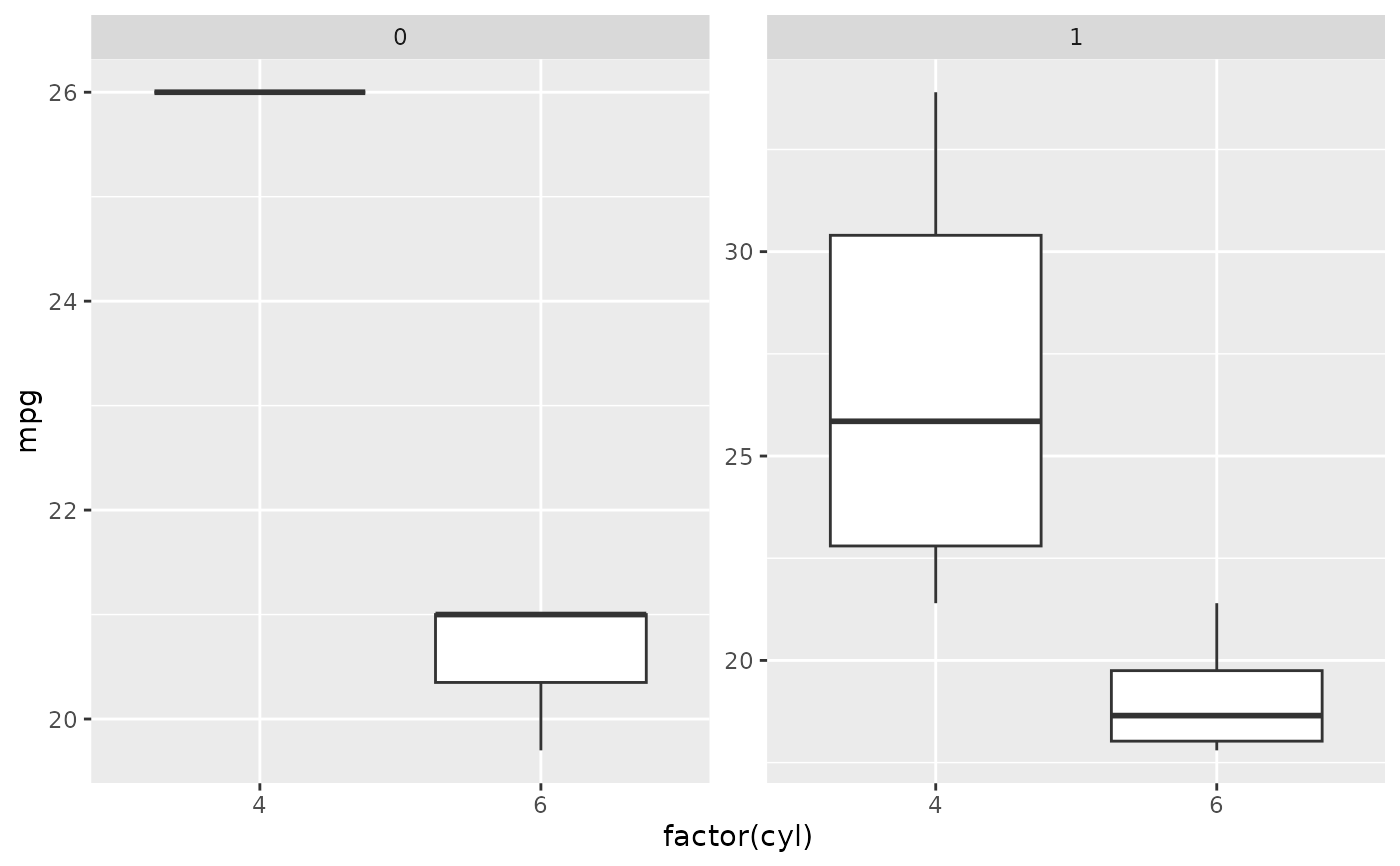This function adds position scales (x and y) of individual panels. These can be used to fine-tune limits, breaks and other scale parameters for individual panels, provided the facet allows free scales.

## Usage

scale_x_facet(expr, ..., type = "continuous")

scale_y_facet(expr, ..., type = "continuous")

## Arguments

expr

An expression that, when evaluated in the facet's layout data.frame, yields a logical vector. See details.

...

Other arguments passed to the scale.

type

A character(1) indicating the type of scale, such that scale_(x/y)_{type} spells a scale function. Defaults to "continuous".

## Value

A scale_facet object that can be added to a plot.

## Details

These scale functions work through the mechanism of the facetted_pos_scales() function, and the same limitations apply: scale transformations are applied after stat transformations, and the oob argument of scales is ignored.

For the expr argument, the expression will be evaluated in the context of the plot's layout. This is an internal data.frame structure that isn't normally exposed, so it requires some extra knowledge. For most facets, the layout describes the panels, with one panel per row. It typically has COL, ROW and PANEL columns that keep track of what panel goes where in a grid of cells. In addition, it contains the facetting variables provided to the facets or rows and cols arguments of the facets. For example, if we have a plot facetted on the var variable with the levels A, B and C, as 1 row and 3 columns, we might target the second B panel with any of these expressions: var == "B", PANEL == 2 or COL == 2. We can inspect the layout structure by using ggplot_build(p)$layout$layout, wherein p is a plot.

When using multiple scale_(x/y)_facet(), the expr argument can target the same panels. In such case, the scales added to the plot first overrule the scales that were added later.

The facetted_pos_scales() function.

## Examples

# A standard plot with continuous scales
p <- ggplot(mtcars, aes(disp, mpg)) +
geom_point() +
facet_wrap(~ cyl, scales = "free")

# Adding a scale for a value for a facetting variable
p + scale_x_facet(cyl == 8, limits = c(200, 600))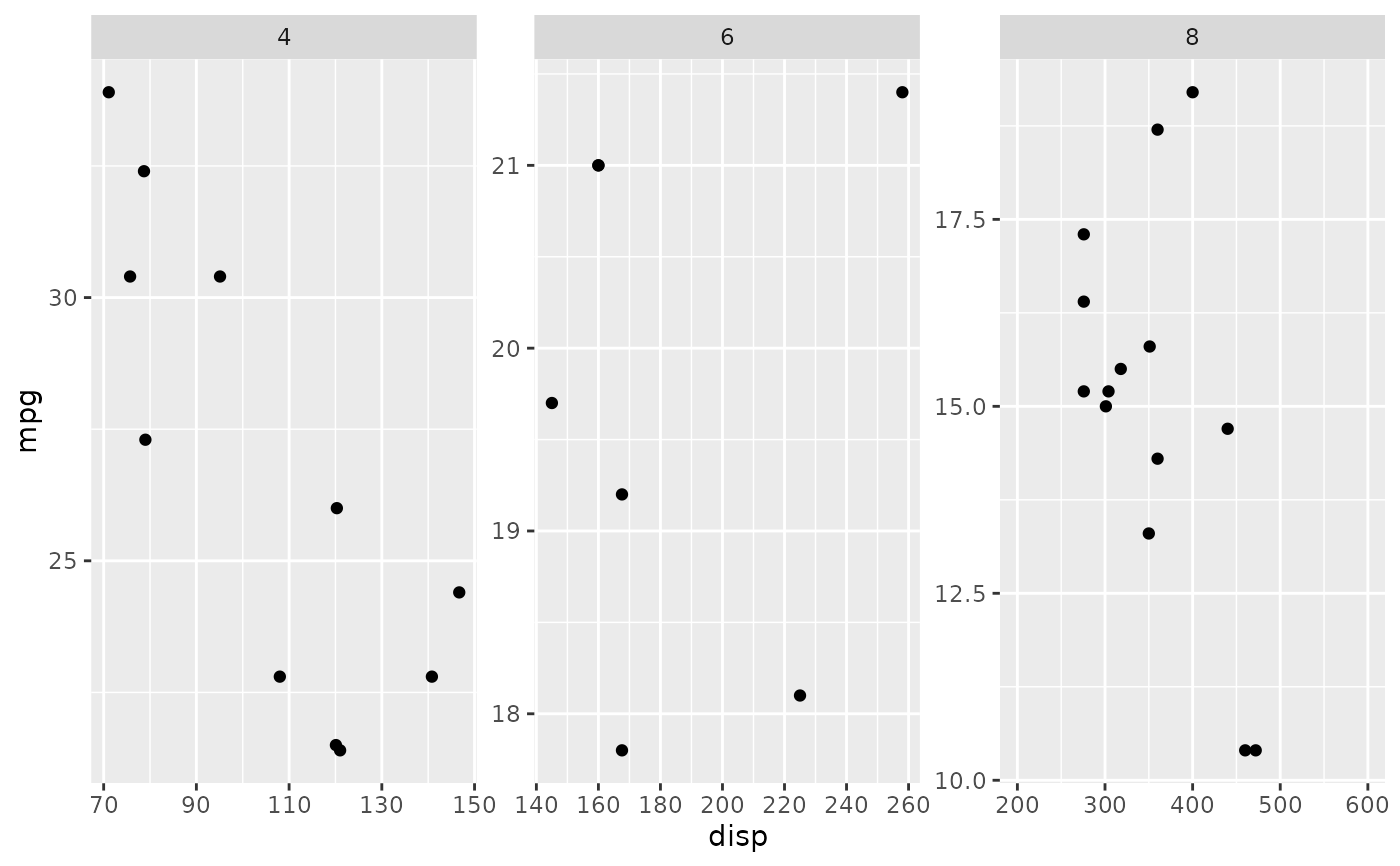# Adding a scale by position in the layout
p + scale_x_facet(COL == 3, limits = c(200, 600))# Setting the default scale and making an exception for one panel
p + scale_y_continuous(limits = c(0, 40)) +
scale_y_facet(PANEL == 1, limits = c(10, 50))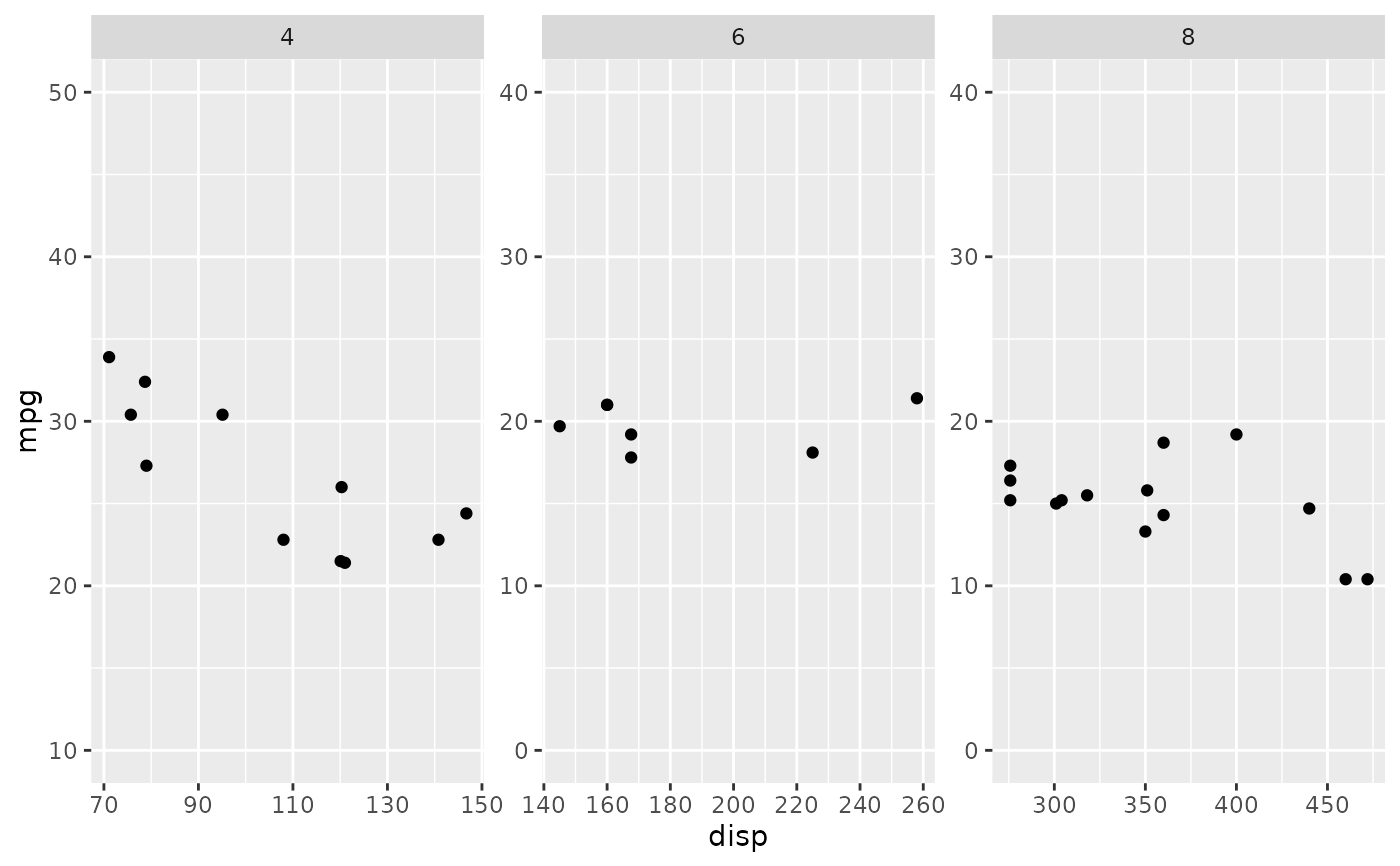# Using multiple panel-specific scales
p + scale_y_facet(PANEL == 1, limits = c(10, 50)) +
scale_y_facet(cyl == 6, breaks = scales::breaks_width(0.5))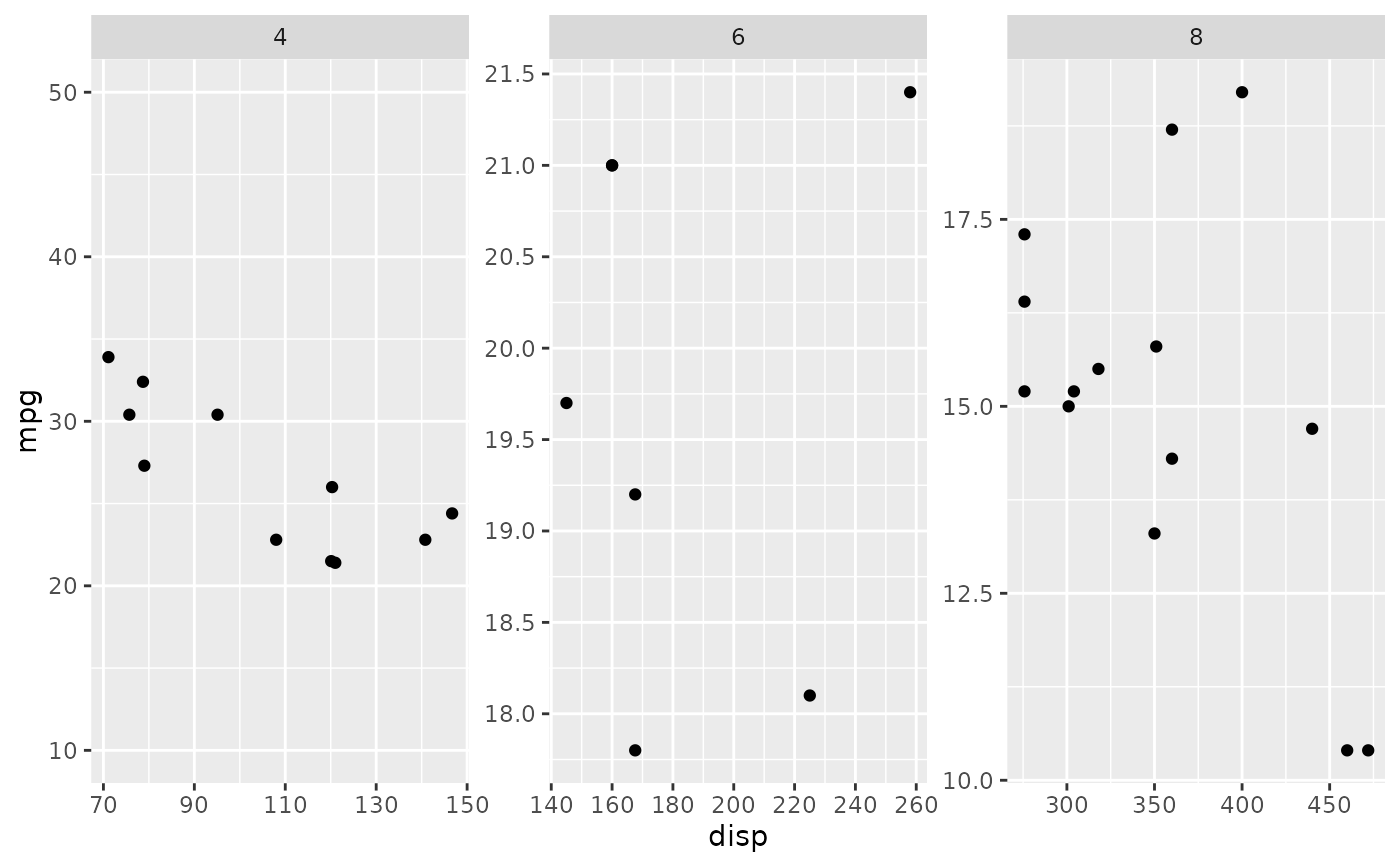# When multiple scales target the same panel, the scale added first gets
# priority over scales added later.
p + scale_y_facet(COL == 2, limits = c(10, 40)) +
scale_y_facet(cyl %in% c(4, 6), breaks = scales::breaks_width(1))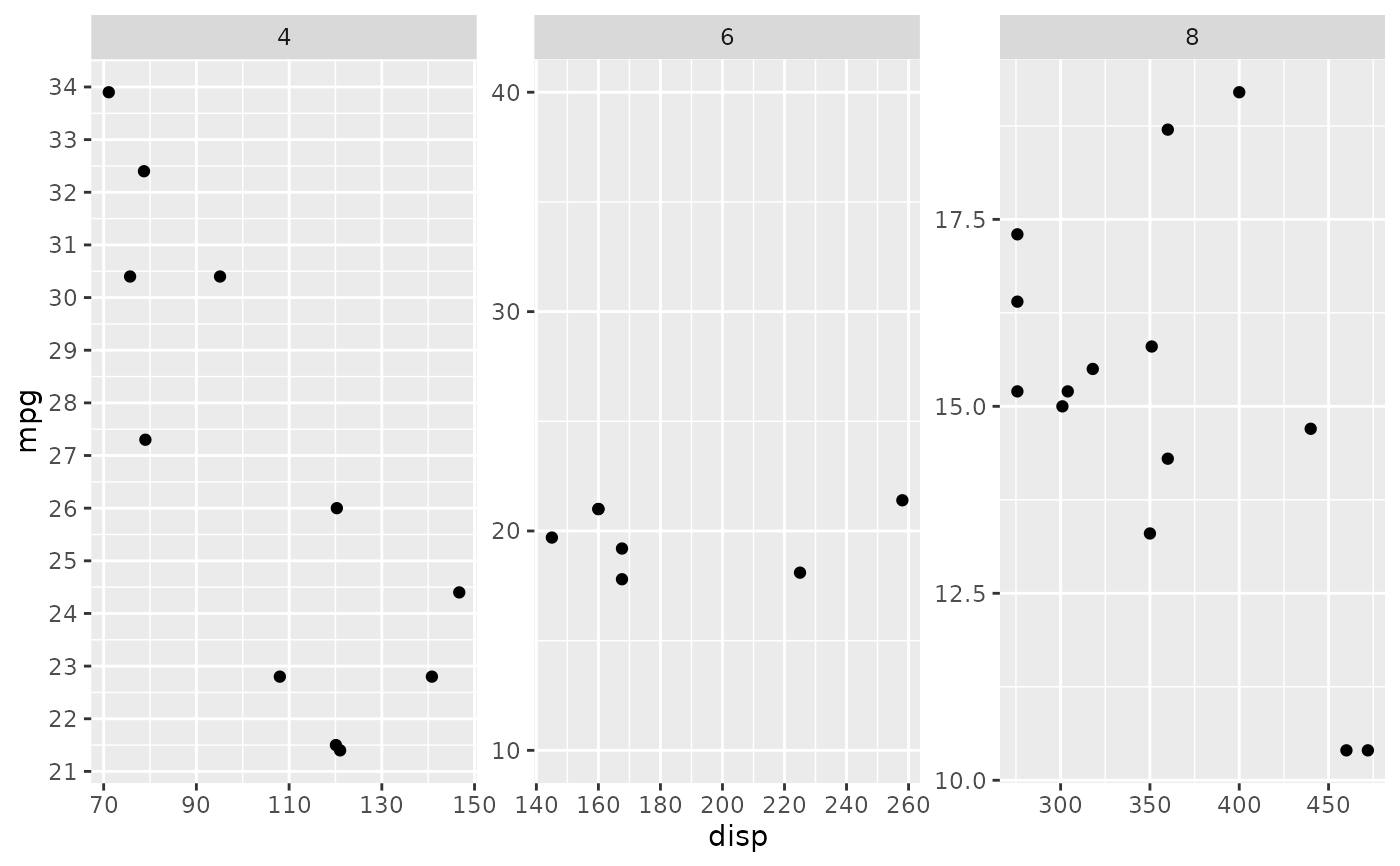# A standard plot with discrete x scales
p <- ggplot(mtcars, aes(factor(cyl), mpg)) +
geom_boxplot() +
facet_wrap(~ vs, scales = "free")

# Expanding limits to show every level
p + scale_x_facet(vs == 1, limits = factor(c(4, 6, 8)), type = "discrete")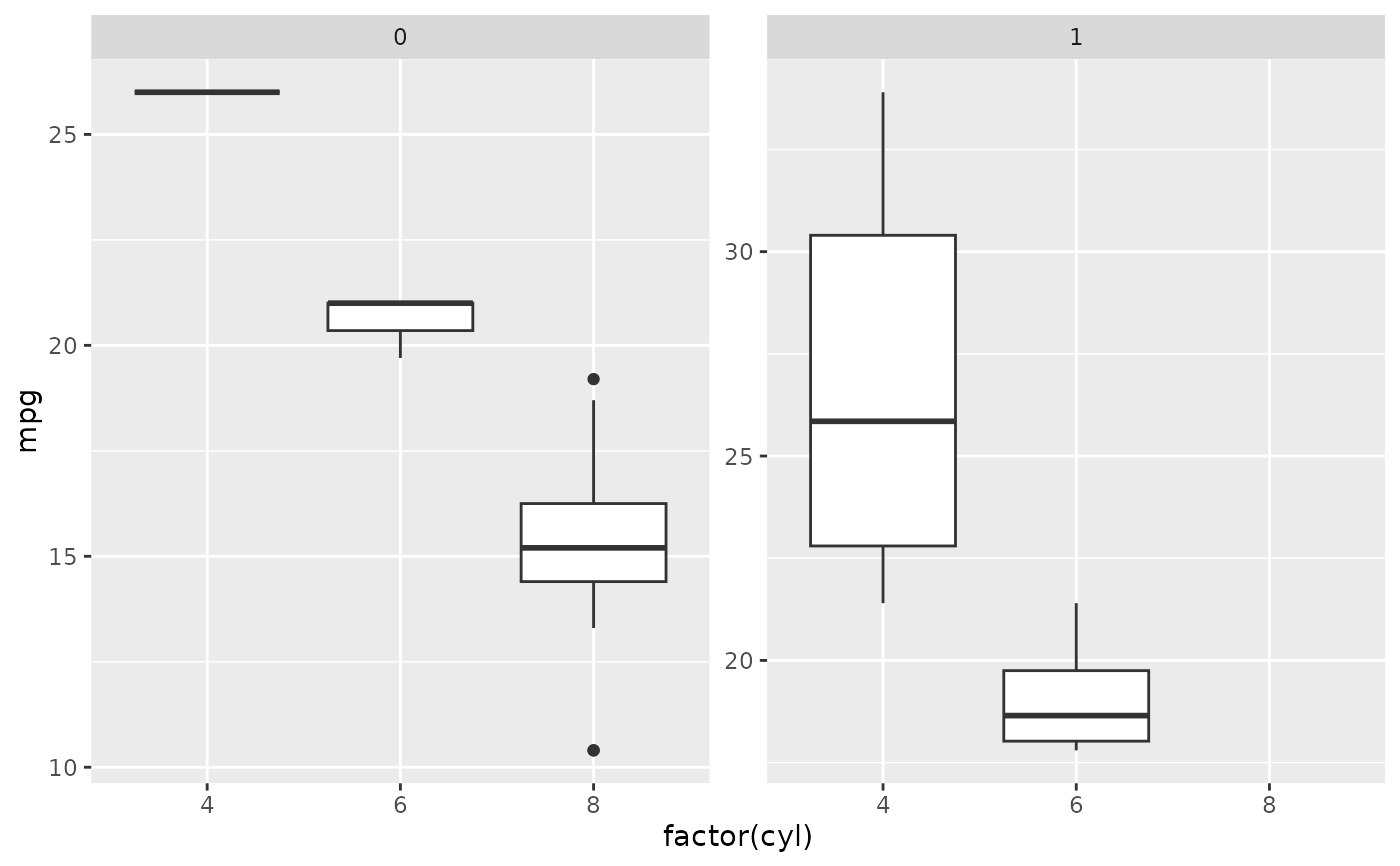# Shrinking limits to hide a level
p + scale_x_facet(vs == 0, limits = factor(c(4, 6)), type = "discrete")
#> Warning: Removed 14 rows containing missing values (stat_boxplot()).